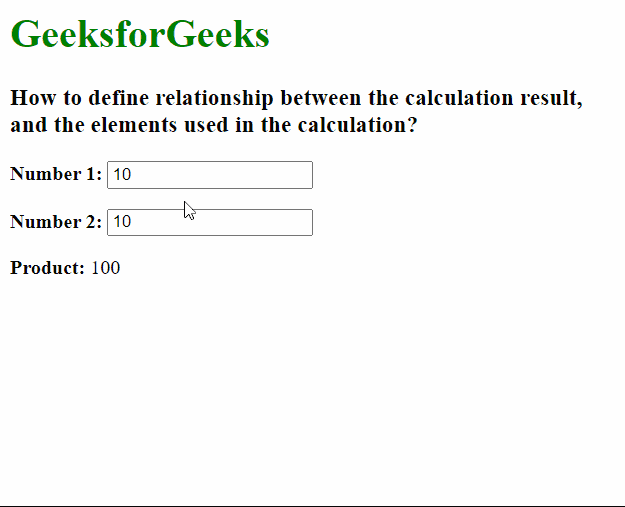Open In App

# How to define relationship between the result and the elements used in the calculation ?

In this article, we will learn how to define a relationship between the elements and the result of a calculation. The <output> element in HTML is a container element that can be used to display the output of a resulting calculation. The site can inject the result in this element. We will use this tag to specify the result.

The form attribute of the output element is used to associate the elements that would be used for the calculation with the output. The value of this attribute must be the id of an <form> in the same document. When this attribute is not specified, it assumes the ancestor <form> element to be used instead. The for attribute is used to denote the id of the elements that contributed to the output.

Example: The below example demonstrates the use of the <output> element.

## HTML

 `<``html``>` `<``body``>``    ``<``h1` `style``=``"color: green"``>GeeksforGeeks``    ``<``h3``>``        ``How to define relationship between the``        ``calculation result, and the``        ``elements used in the calculation?``    ````    ````    ``<``form` `oninput``=``            ``"val.value = parseInt(num1.value) * parseInt(num2.value)"``>``        ``` `        ``<``p``><``b``>Number 1:``            ``<``input` `type``=``"number"` `id``=``"num1"` `value``=``"10"` `/>``        ````        ``<``p``><``b``>Number 2:``            ``<``input` `type``=``"number"` `id``=``"num2"` `value``=``"10"` `/>``        ``` `        ````        ``<``p``>``            ``<``b``>Product:``            ``<``output` `name``=``"val"` `for``=``"num1 num2"``> 100 ``        ````    ````` ``

Output: